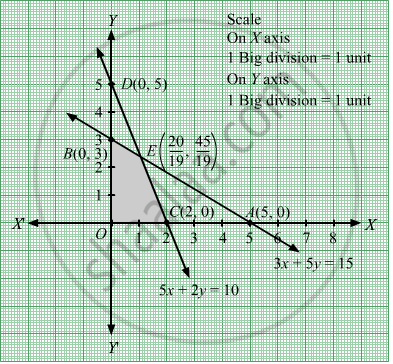# Maximize Z = 5x + 3y Subject to 3 X + 5 Y ≤ 15 5 X + 2 Y ≤ 10 X , Y ≥ 0 - Mathematics

Sum

Maximize Z = 5x + 3y
Subject to

$3x + 5y \leq 15$
$5x + 2y \leq 10$
$x, y \geq 0$

#### Solution

First, we will convert the given inequations into equations, we obtain the following equations:
3x + 5y = 15, 5x + 2y = 10, x = 0 and y = 0
Region represented by 3x + 5y ≤ 15 :
The line 3x + 5y = 15 meets the coordinate axes at A(5,0) and B(0,3) respectively. By joining these points we obtain the line 3x + 5y = 15.
Clearly (0,0) satisfies the inequation 3x + 5y ≤ 15. So,the region containing the origin represents the solution set of the inequation 3x + 5y ≤ 15.
Region represented by 5x + 2y ≤ 10 :
The line 5x + 2y = 10 meets the coordinate axes at C(2,0) and D(0,5) respectively. By joining these points we obtain the line 5x + 2y = 10.
Clearly (0,0) satisfies the inequation 5x + 2y ≤ 10. So,the region containing the origin represents the solution set of the inequation 5x + 2y ≤ 10.
Region represented by x ≥ 0 and y ≥ 0:
Since, every point in the first quadrant satisfies these inequations. So, the first quadrant is the region represented by the inequations x ≥ 0, and ≥ 0.The feasible region determined by the system of constraints, 3x + 5y ≤ 15, 5x + 2y ≤ 10, x ≥ 0, and y ≥ 0, are as follows.The corner points of the feasible region are O(0, 0), C(2, 0),
$E\left( \frac{20}{19}, \frac{45}{19} \right)$ and B(0, 3).

The values of Z at these corner points are as follows.

 Corner point Z = 5x + 3y O(0, 0) 5 × 0 + 3 × 0 = 0 C(2, 0) 5 × 2 + 3 × 0 = 10 $E\left( \frac{20}{19}, \frac{45}{19} \right)$ 5x $\frac{20}{19}$ +3x $\frac{45}{19}$ = $\frac{235}{19}$ B(0, 3) 5  0 + 3 × 3 = 9

Therefore, the maximum value of Z is

$\frac{235}{19}\text{ at the point } \left( \frac{20}{19}, \frac{45}{19} \right)$ .Hence, x= $\frac{20}{19}$  and y =
$\frac{45}{19}$  is the optimal solution of the given LPP.
Thus, the optimal value of Z is $\frac{235}{19}$ .
Concept: Graphical Method of Solving Linear Programming Problems
Is there an error in this question or solution?

#### APPEARS IN

RD Sharma Class 12 Maths
Chapter 30 Linear programming
Exercise 30.2 | Q 1 | Page 32